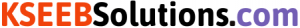# KSEEB Solutions for Class 6 Maths Chapter 3 Playing with Numbers Ex 3.6

Students can download Chapter 3 Playing with Numbers Ex 3.6 KSEEB Solutions for Class 6 Maths helps you to revise the complete syllabus.

## Karnataka State Syllabus Class 6 Maths Chapter 3 Playing with Numbers Ex 3.6

Question 1.
Find the HCF of the following numbers:
(a) 18, 48
(b) 30,42
(c) 18, 60
(d) 27, 63
(e) 36, 84
(f) 34, 102
(g) 70, 105, 175
(h) 91, 112, 49
(i) 18, 54, 81
(j) 12, 45, 75
Solution:
a) 18, 48
18 = 1, 2, 3, 6, 9, 18
48 = 1, 2, 3, 4, 6, 8, 12, 16, 24, 48
Common factors = 1, 2, 3, 6
H.C.F of 18 & 48 = 6

b) 30, 42
30 = 1, 2, 3, 5, 6, 10, 15, 30
42 = 1, 2, 3, 4, 6, 7, 14, 21, 42
Common factors = 1, 2, 3, 6
∴ H.C.F. = 6
∴ H.C.F of 30 & 42 is 6c) 18 & 60
18 = 1, 2, 3, 6, 9, 18
60 = 1, 2, 3, 4, 6, 10, 12, 15, 30, 60
Common factors = 1, 2, 3, 6
∴ H.C.F. of 18 & 60 is 6

d) 27 & 63
27 = 1, 3, 9, 27
63 = 1, 3, 7, 9, 21, 63
∴ Common factors = 1, 3, 9
∴ H.C.F. of 27 & 63 is = 9.

e) 36 & 84
36 = 1, 2, 3, 4, 6, 9, 12, 18, 36
84 = 1, 2, 3, 4, 6, 7, 12, 14, 21, 28, 42, 84
∴ Common factors = 1, 2, 3, 4, 6, 12
∴ H.C.F. of 36 & 84 is 12.

f) 34 & 102
34 = 1, 2, 17, 34
102 = 1, 2, 3, 6, 17, 34, 51, 102
∴ Common factors = 1, 2, 17, 34
∴ H.C.F. of 34 & 102 is 34.

g) 70, 105, 175
70 = 1, 2, 5, 7, 10, 14, 35, 70
105 = 1, 3, 5, 7, 10, 14, 35, 105
175 = 1, 5, 7, 25, 35, 175
∴ Common factors 1, 5, 7, 35
∴ H.C.F. of 70, 105, 175 is 35

h) 91, 112, 49
91 = 1, 7, 13, 91
112 = 1, 2, 4, 7, 8, 14, 16, 28, 56, 112
49 = 1, 7, 49
∴ Common factors = 1, 7
∴ H.C.F. of 91, 112, 49 is 7i) 12, 45, 75
12 = 1, 2, 3, 4, 6, 12
45 = 1, 3, 5, 9, 15, 45
75 = 1, 3, 5, 15, 26, 75
∴ Common factors 1, 3
∴ H.C.F of 12, 45, 75 is 3.

Again, all the prime numbers you used to divide above are the Prime Factors of 70.

Question 2.
What is the HCF of two consecutive
(a) numbers?
(b) even numbers?
(c) odd numbers?
Find the HCF of the following:
(i) 24 and 36
(ii) 15, 25 and 30
(iii) 8 and 12
(iv) 12, 16 and 28
Solution:
(i) 1 e.g., HCF of 2 and 3 is 1.
(ii) 2 e.g., HCF of 2 and 4 is 2.
(iii) 1 e.g., HCF of 3 and 5 is 1.Factors of 84 are the numbers that can divide 84 and leaves a remainder zero.

Question 3.
HCF of co-prime numbers 4 and 15 was found as follows by factorisation:
4 = 2 × 2 and 15 = 3 × 5 since there is no common prime factor, so HCF of 4 and 15 is 0. Is the answer correct? If not, what is the correct HCF?
Solution:
No. The answer is not correct. 1 is the correct HCF.